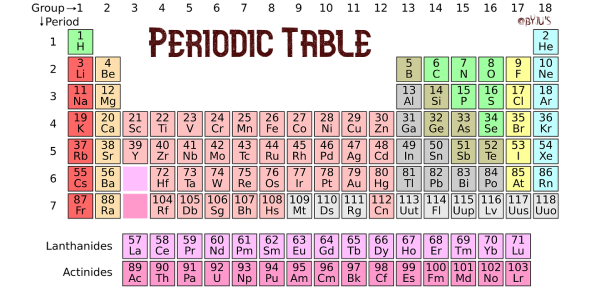# Periodic Table: Chemistry Trivia Quiz!

10 Questions | Total Attempts: 843SettingsThe periodic table is a tabular display of the chemical elements which show the atomic number, electron configuration, and chemical properties. There are 118 elements in the periodic table. Take this Chemistry Trivia Quiz to test your knowledge and learn about the elements. All the best!

• 1.
The symbol of gold is
• A.

Au

• B.

Ag

• C.

Ge

• D.

Ga

• 2.
The pH of water is
• A.

0

• B.

1

• C.

14

• D.

7

• 3.
Which among the following is not a noble element?
• A.

He

• B.

Kr

• C.

K

• D.

Ne

• 4.
Boiling point of water is
• A.

273 K

• B.

283 K

• C.

298 K

• D.

0 C

• 5.
There are ______  types of blocks of elements in Periodic Elements.
• 6.
Sodium atom is bigger than Potassium.
• A.

True

• B.

False

• 7.
The pH of rainwater is 5.6.
• A.

True

• B.

False

• 8.
Freezing of water is an endothermic reaction.
• A.

True

• B.

False

• 9.
A solution of pH value 1 is
• A.

Weak base

• B.

Strong acid

• C.

Strong base

• D.

Weak acid

Related Topics